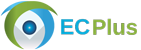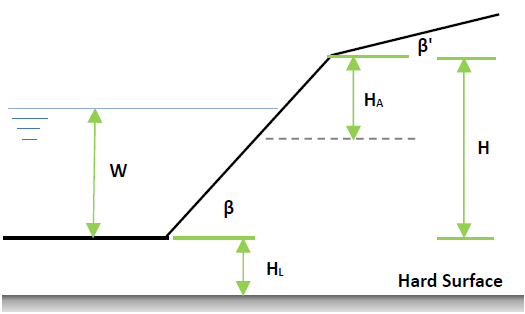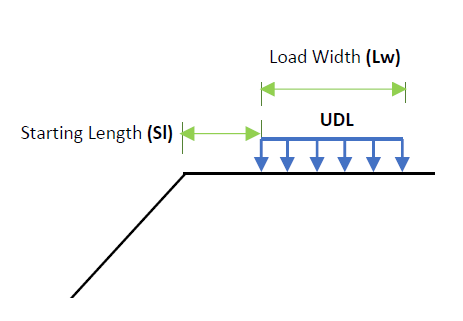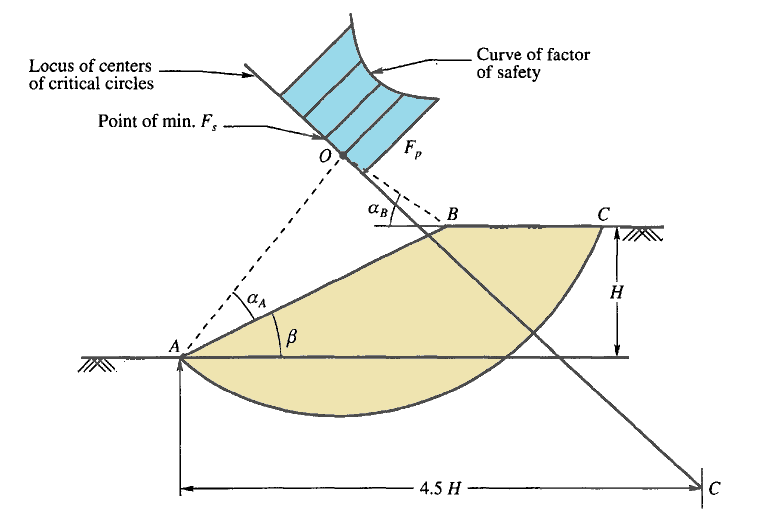# Slope Stability Analysis DesignSample Report

Slope stability analysis is to mathematically evaluate the stability of an embankment such as earth dam or natural slope. ECPLUS adopts the widely used Fellanius Slip Circle method to determine the factor of safety a slope against possible sliding.

The stability of a slope is affected by the following:
1. Height of the slope
2. Properties of the soil fill
3. Presence of water
4. Pore Pressure

## Features

• The following features are available for the slope stability analysis
• Units
• SI Unit (kN, m, mm)
• Imperial Unit (kips, ft, in)
• Slope Geometry
• Option to define the top surface slope
• Water level
• Presence of Hard Layer (Firm Base)
• One or two types of soil fill layers• Surcharge loads on top surface
• Surcharge distributed loads with finite or infinite width• Stability Analysis
• User Defined Slip Circle
• Auto Stability Analysis - Program determined critical slip circleThe auto detection of critical slip circle is depicted above. Where αA and αB are predefined angles as per slope inclination β

## Design Considerations

• Each slice is considered on its own and interactions between slices are neglected because the resultant forces are parallel to the base of each slice
• It is assumed that the boundary between soil types is horizontal and above the toe of slope
• Water level when specified, slope may be submerged in water retaining embankment such as earth dam or canal bank
• Hard surface (Firm Base) when specified beneath the base, it is assumed to constrain the depth of any slip circle
• It is assumed that each slice can rotate about a centre of rotation and that moment balance about this point is also needed for equilibrium
• Pore pressure ratio is assumed constant for the entire slope

## Revision

• Ver 1.0 - Original versionSample Report